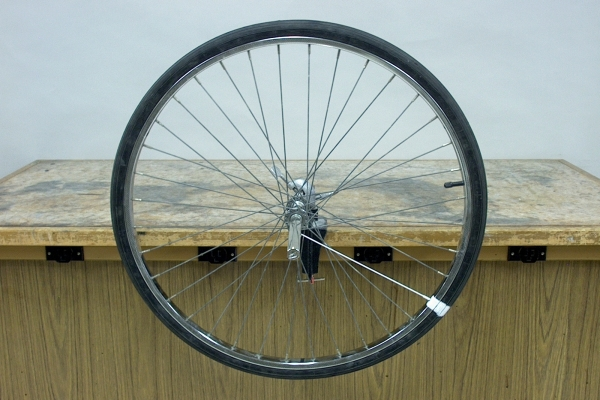A video of this demonstration is available at this link.

You can illustrate the variables of rotational motion with this bicycle wheel.

The demonstrations in section 08 -- Kinematics, allow you to show straight-line motion at constant velocity, accelerated straight-line motion and linear motion in two dimensions. With their aid, you can illustrate the concepts of position, (linear) velocity and (linear) acceleration, and derive the equations of motion in a straight line and in a plane. (Two of the demonstrations in section 04 -- Measurement, allow you to show the Cartesian coordinate system and vectors, which are essential to any discussion of the aforementioned concepts.) In similar fashion, this demonstration allows you to show rotational motion, that is, motion in which an object rotates about a particular axis. If we take the x-axis as the horizontal line that passes through the center of the wheel, and the y-axis as the vertical line passing through the center of the wheel, then the axle of the wheel points along the z-axis. The wheel, of course, rotates about the z-axis. A white stripe on the rim serves as a reference for the position of the wheel.

Whereas in linear motion, we denote a position along the x- or y-axis (or both) with x and y coordinates, in rotational motion, we describe position in terms of an angular displacement with respect to a reference position, say the x-axis. By convention, the positive direction is counterclockwise, and the angle, θ, equals s/r, where r is the radius of the circle along which the point on the object moves, and s is the length of the arc from zero to its present position. So, taking the positive x-axis as the zero reference, the white stripe on the wheel in the photograph above is at an angular position of about 5.5 radians (≈ 315°).

In what follows, we consider the wheel as a rigid body, that is, we assume that all points on the wheel maintain the same spatial relationship to each other as the wheel rotates.

If the the wheel is rotating at constant speed, its change in position with respect to time is a change in angle with respect to time, Δθt. This is its average angular speed. The instantaneous angular speed is the same ratio in the limit as Δt goes to zero, usually denoted as ω; ω = /dt. This is also the magnitude of ω (= dθ/dt), the angular velocity, which points along the axle of the wheel. If the wheel is rotating in the counterclockwise direction, then according to the right-hand rule, ω points outwards, away from the table. The units of ω are typically rad/s. Depending on context, one also sees rev/s or deg/s.

If the speed of rotation of the wheel is not constant, then the wheel is accelerating, and ω is changing with time. As with angular speed, the average angular acceleration is Δωt, and, in the limit as Δt goes to zero, the instantaneous angular acceleration, α, equals /dt. Its units are typically rad/s2, but depending on context, you might also see rev/s2 or deg/s2. This is also a scalar quantity that corresponds to the magnitude of a vector, α, which equals dω/dt. As for ω, α points according to the right-hand rule. If we accelerate the wheel in our example in the counterclockwise direction, so that its speed is constantly increasing in that direction, then α points outwards, away from the table.

Our earlier statement about rigid bodies means that both ω and α are the same for all points on the wheel (or other rotating object).

The scalar quantities θ, ω (= /dt) and α (= /dt = d2θ/dt2) are analogous to the scalar quantities x, v (= dx/dt) and a (= dv/dt = d2x/dt2), respectively, and the vector quantities θ, ω (= dθ/dt) and α (= dω/dt = d2θ/dt2) are analogous to the vector quantities r, v (= dr/dt) and a (= dv/dt = d2r/dt2), respectively. This leads to a set of equations that are analogous to those for linear motion (given here in scalar form, assuming motion in the x direction and that x0 = 0):

 Linear motion Rotational motion v = v0 + at ω = ω0 + αt x = (1/2)(v0 + v)t θ = (1/2)(ω0 + ω)t x = v0t + (1/2)at2 θ = ω0t + (1/2)αt2 v2 = v02 + 2ax ω2 = ω02 + 2αθ

We should also note that when the wheel is rotating at a constant speed, though all points on the wheel have the same angular speed, ω, their instantaneous linear speeds, in the direction tangent to the circles in which they are rotating, are proportional to their distance from the axis of rotation. If we call this instantaneous linear speed v, for a point at a distance r from the axis of the wheel, v = ωr. Similarly, though all points have the same angular acceleration, their instantaneous linear accelerations (again tangential to the circle of motion) are proportional to their distance from the axis of rotation, or a = αr.

References:

1) Resnick, Robert and Halliday, David. Physics, Part One, Third Edition (New York: John Wiley and Sons, 1977), pp. 32, 215-223.